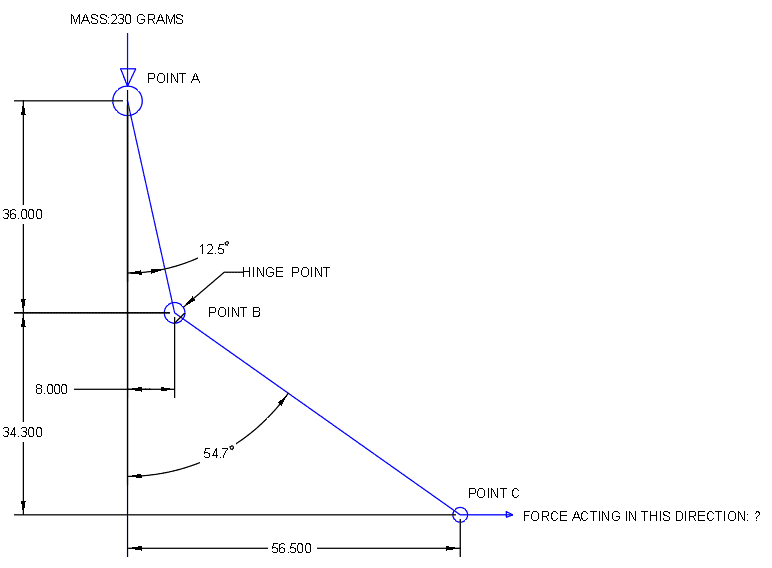# How to calculate the Resultant force due to a Lever arm?

JPakt
Homework Statement:
Need help to find the resultant force at the Point "C" on an object, when a 230-gram force applied at Point "A" which is hinged at point "B".
Relevant Equations:
F1.x1 = F2.x2Homework Helper
Gold Member
2022 Award
The set up needs more description.
Does the blue line represent a rigid, but bent, arm, or two arms hinged independently at B?
Is B free to move around in space or constrained somehow?
Are there constraints on A's motion?

Also, you need to post an attempt, per forum rules.

•JPakt and gneill
JPakt

- 2 Blue Line represents a rigid body
- Point B is hinge support which allows the rotation in an anticlockwise direction and applies force in the direction highlighted at point "C" on a spring.
- Point A represents the CG of the body

Please find the attachment which illustrates my attempt to solve the problem.

#### Attachments

Homework Helper
Gold Member
2022 Award
Yes, thank you.
The mass is 230gm. That is a mass, not a force, which makes your equations for forces incomplete.
Other than that, your method works, but there is an easier way. You do not need to find F1 to find the torque exerted by the weight. Likewise at the other end.

JPakt